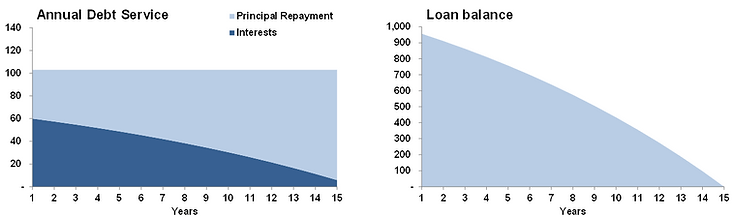top of page

## Financing

Debt repayment

To finance its development, a company or a project may decide to raise debt. This can be done in the form of a bank loan or through issuing bonds on the debt capital markets. Modeling bonds is quite straightforward as in the vast majority of the cases, the face value of the bond is repaid only at maturity and only interests are paid until then.

Bank loans can be repaid through various methodologies but the two most common loan amortization methods used are i) mortgage style and ii) linear.

Mortage style

This amortization methodology assumes that the debt service is constant through the lifetime of the loan, this means that the sum of interest and principal repayment that have to be paid periodically is constant over time, although the split between interest payment and principal repayment changes over time.

Excel can calculate the annuity (the sum of principal repayment and interest payment) with the PMT formula.

Its syntax is the following:=PMT(Interest Rate,Number of periods of repayment,Amount to be repaid)

Example 1: for a loan amount of 1,000, a maturity of 15 years with annual instalments and a 6% interest rate, the formula is

PMT(6% , 15 , 1000)

Example 2: for a loan amount of 1,000, a maturity of 15 years with semi-annual instalments and a 6% interest rate, the formula is

PMT(3% , 30 , 1000)

As you may have noticed in example 2, since instalments are semi-annual there are 30 instalments and the interest rate has been halved (the interest rate entered must be consistent with the periocidity of the instalments, if 6% is the annual interest rate, then the interest rate for 6 months is 3%).

Excel modeling

1. Calculate the annuity using the PMT formula

2. For each period, calculate the interests to pay from the opening balance (interest rate x opening balance of the loan for this period)

3. To find the principal amount to repay, calculate the difference between the annuity and the interests (since the sum of principal repayment + interest payment is always equal to the annuity):

Principal Repayment=Annuity - Interests

4. To make sure the repayment stops at some point, make sure that the amount to be repaid is the minimum between the opening balance (the amount that remains to be repaid) and the Principal Repayment as calculated in 3.

Below is a graphical representation of the debt service (on the left) and the remaing loan balance (on the right) using a mortgage style amortization. As you can see, even though the sum of principal repayment and interests is always constant over time, the breakdown between the two changes over time.Linear

This amortization methodology assumes that the amount of principal repaid over time is the same for each period.

To calculate the amount of principal repayment you have to divide the initial loan amount by the number of instalments.

Example 1: for a loan amount of 1,000, a maturity of 15 years with annual instalments and a 6% interest rate, an amount equal to 1,000/15=66.67 will be repaid at each instalment.

Example 2: for a loan amount of 1,000, a maturity of 15 years with semi-annual instalments and a 6% interest rate, an amount equal to 1,000/30=33.33 will be repaid at each instalment.

Because the principal is repaid more quickly than in the Mortgage style amortization, interest payment also drop more quickly. The drawback is that the debt service in the first years is higher than in the Mortgage style amortization.

Excel modeling

1. Calculate the amount of principal to be repaid at each instalment by dividing the loan amount by the number of instalments

2. For each period, calculate the interests to pay from the opening balance (interest rate x opening balance of the loan for this period)

3. To make sure the repayment stops at some point, make sure that the amount to be repaid is the minimum between the opening balance (the amount that remains to be repaid) and the Principal Repayment as calculated in 1.

Below is a graphical representation of the debt service (on the left) and the remaing loan balance (on the right) using a linear amortization. As you can see, since the same amount of principal is repaid at every instalment, both the loan balance and the debt service decline linearly.Comparison

The mortgage style amortization offers a lower debt service in the early years of the loan while the linear amortization will require lower overall interest payments as the principal is repaid more quickly.bottom of page